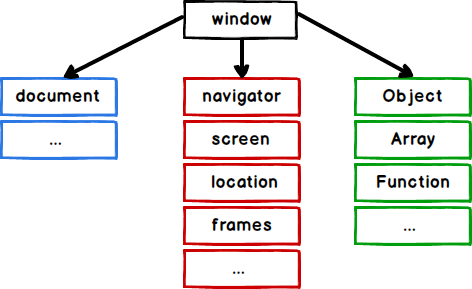MCQ

# JavaScript MCQs – Multiple Choice Questions and Answers – Part 2

Multiple choice questions and answers (MCQs) on JavaScript to prepare for exams, tests, and certifications. These questions are taken from a real written exam and some parts are taken from an interview. So you will find questions on basic techniques such as variables, functions, loops, objects, and more. This quiz will easily prepare anyone to pass their online test.

###### 1. What is the object that is in TOP of the root in JavaScript?

A url

B top

C window

D document

C###### 2. How to insert a comment on several lines?

A

```//This comment has
more than one line //```

B

C

```/* This comment has
more than one line  */```

D All the answers are true

C

###### 3. How do you find the greatest value of “a” and “b”?

A Math.ceil(a, b)

B Math.max(a, b)

C ceil(a, b)

D top(a, b)

B

###### 4. How can you add a comment in JavaScript code?

A `//This is a comment`

B `"This is a comment"`

C

D `#This is a comment`

A

A True

B False

B

###### 6. How to round the number 3.12 to a closer integer number?

A `Math.round(3.12)`

B `Math.rnd(3.12)`

C `float(3.12)`

D `Math.float(3.12)`

A

###### 7. What is the correct syntax of the “while” loop?

A `while (i <= 5)`

B `while i = 1 to 5`

C `while (i <= 5; i++)`

D `while (i=0; i <= 5; i++)`

A

###### 8. What is the correct syntax of the “for” loop?

A `for (i <= 10; i++)`

B `for i = 1 to 10`

C `for (i = 0; i <= 10)`

D `for (i = 0; i <= 10; i++)`

D

###### 9. What is the correct syntax for opening a new window called “f2”?

A `f2 = window.new("https://stackhowto.com");`

B `f2 = window.open("https://stackhowto.com");`

C `f2 = document.open("https://stackhowto.com");`

D `f2 = document.new("https://stackhowto.com");`

B

###### 10. What is the correct syntax to write an array in JavaScript?

A `var fruits = 1 = ("orange"), 2 = ("banana"), 3 = ("apple")`

B `var fruits = (1:"orange", 2:"banana", 3:"apple")`

C `var fruits = "orange", "banana", "apple"`

D `var fruits = ["orange", "banana", "apple"]`

DMCQPractice competitive and technical Multiple Choice Questions and Answers (MCQs) with simple and logical explanations to prepare for tests and interviews.Read More